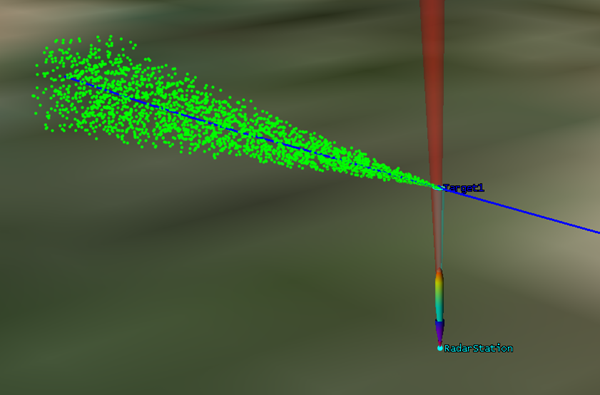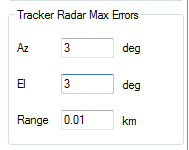Back

# Realistic Radar-Generated Tracks in STK

I had many customers asking whether STK can generate realistic radar tracks. Hence I plan to develop an application, which develops tracks taking into account the radar performance metrics generated by STK.

In STK, radar performance metrics generated is based on the mathematical configuration of the radar and the RCS of the target object. In reality, there will be several other unknown glitches, which leads to the wrong geolocation of the detected targets. Errors in a track generated by a radar, considering various unknown error sources could be the summarized into the following:

• Error in Azimuth angle to the target
• Error in Elevation angle to the target
• Error in Range to the target

These unknown errors could be the results of hardware calibration requirements, weather conditions, deterioration of performance with age of the radar etc.

In STK, the efficiency of a radar to detect an object is reflected in the ‘Probability of Detection’ value. We will be using this value as well as the above three unknown errors to detect the total errors expected in the geolocation of the target. The equations used are given below. These calculated errors are then added to the original location of the target. A random factor is included in the equation to account for the randomness of the unknown errors. The below given equations shows the calculation of radar generated Azimuth, Elevation and Range direction to the target.

Similarly, we use the following equations for Elevation and Range

Using these Az, El & Range values, the LLA tracks are generated using STK-Analysis Work Bench.

Figure 1 shows a track produced by using the method described above. A tracker radar detects the PDet on a target and the position “as generated by the radar” is shown in green dots in the figure below. The blue line is the original track.Figure 1: Radar generated tracks shown as green dots

The following max errors were supplied in this case:As you can see from Figure 1, as the target approaches the radar, the location error reduces significantly. This is because the PDet value approaches towards 1. The results can be used to evaluate the performance of the radar and to test the filtering schemes (e.g. kalman filter) on the generated tracks.

The below given video shows how the tracks are generated in real-time in STK.